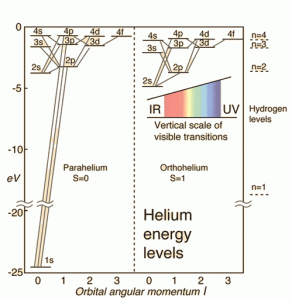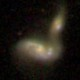# The Illusion Of Truth

In Science by Brian Koberlein6 Comments

Physical theories are often presented as a description of what’s really going on. Forces act on a baseball, causing it to fall. Atoms collide and fuse in the heart of a star, releasing heat and energy. Science is true, as is often said, and our scientific theories encapsulate this truth. But this isn’t entirely true.

The probability distributions for hydrogen in different energy states.

For example, quantum mechanics is a strange, sometimes confusing theory. Objects can be particles and waves. They do strange things like tunnel through barriers, and can even appear and disappear.  Given all this strangeness, what’s really going on? What’s the true nature of quantum reality? It depends on which approach you want to take. In one view quantum objects are described by a wave-like probability function. When you interact with or “observe” the object this “wavefunction” collapses into a definite state. That’s view commonly presented, but a quantum system can also be described by its transitions between energy states. Since this uses a mathematical method involving matrices, it’s known as matrix mechanics. Both wavefunctions and matrix mechanics give the same results, but their view of what really goes on is very different. Then there’s the path integral method. Rather than a wavefunction or matrix transitions, path integrals imagine quantum objects can take almost any path between two states. By summing all the possible paths you can derive the odds that it will occur.The matrix transition version of quantum theory doesn’t care about the wavefunction.

So which one is true? Are quantum objects distributed waves of probability? Are they simply transitions between energy states? Do they take an infinite number of paths between states? Each of these models make the same predictions, so one version is no more “true” than the others. What happens in practice is that we’ll use whatever method is useful at the time. They are equivalent models, so the best model for the job is the one we’ll use. The only reason the wavefunction view is so common is that it’s the version usually taught to introductory students.

You might think this uncertainty of truth is due to the behavior of quantum physics itself. It’s so strange and counterintuitive that we can’t wrap our puny brains around what’s really going on. But the same thing occurs in lots of other fields. Even something as straight forward as basic Newtonian physics.

Toss a baseball in the air and Earth’s gravitational force pulls it down. The force of gravity is a simple truth, right? While we often describe classical motion in terms of forces and acceleration, we can also describe it in terms of energy and momentum. In the Lagrangian and Hamiltonian approach, the path of a baseball is the optimized path among possibilities. In this view a baseball’s path is the extrema of an energy equation, and force can be derived as a necessary consequence of this. In the relativistic view the baseball follows a geodesic, which is the minimal path through space and time. So is a baseball’s motion due to a gravitational force, an energy extrema, or a spacetime geodesic? As with quantum theory, different approaches yield the same result. They are mathematically equivalent, so we can use whatever method is most useful at the time.

At its core, science is less about truth and more about models. The metaphysics underlying a model is useful only as far as it allows us to make better predictions, generate new ideas, or bring models together as a cohesive whole. This is why we have no problem using classical gravity to calculate the path of a spacecraft through the solar system, while using special relativity to account for the Doppler shift of the spacecraft’s radio signals. It’s why we can use quantum physics to study atoms in the morning, and general relativity to study black holes in the afternoon. In regimes where models conflict with each other it isn’t a failure of truth, but instead shows an opportunity to develop a better model.

It could be that with each better model we move closer to the truth about reality. The truth is out there, and science strives to move towards that truth. It’s a common view, and certainly the search for truth has driven many scientists to develop better and better models. But the real power of science is the recognition that what we have are models. Our models can be powerful, but they are always a bit tentative. There’s always a chance that they might just be an illusion of truth.

1.what is shown in the main image?
I am confused from the three models you describe:
1. wave model that transitions to object model when the observer adds ‘certainty’ to the observation
2. matrix model looks like animation- it relies on stuff constantly moving which assumes that we have a wave/closer to object/ that propagates in linear fashion. From what you describe the object here is a vector of states and although practically considered an object it is computed as a wave that is composed of separate waves, that are synchronized somehow so that a vector can be built from those.
3. the integral path model looks like we have anti-waves. It still focuses on the dual-confusion idea for certainty, but it observes the space where that same pseudo-observed/certain/ object as an item positioned on a topological map of potentials that drive it towards one or different direction.

Observing the system from the integral path model objects can teleport(tunnel) since it expects somewhat continuous existence of the pseudo-observed object. Observing the system from the matrix model we see components being created, destroyed and composed. Looking from the wave model we have both the anti-wave topology and the object discretization(is an object at a position, is not an object in a position).
All three approaches require synchronization between the composites(system states, system constructs) over a specific time frame. The latter is imposed even though the time frame at which the system cluster operates is very different from the time frame of the observer. The latter can be recursively applied to the system cluster since the composites sharing different properties(like energy and so speeds and interfaces) posses their own time frames. Given that why do we try to synchronize? Doing quantum phisics is not like using pins to catalogue butterflies. The way I feel it is more like trying to pass the same river twice.

2.If quantum physics depicts impossible physical characteristics on mass-like objects why should quantum space not also be equally strange and irrational? Though it’s equally irrational for a non-physicist to make conjecture but I see no reason to think the space surrounding atoms to be completely different from the space humans live and breathe in. Why shouldn’t quantum objects “behave badly” in that space? I suspect a better question might be, “Can we “large objects” take advantage of quantum space and use it to our advantage?”

3.Brian. It is perhaps not that the models and results are an illusion of truth but rather that truth itself is an illusion, i.e. truth is just a colloquial term for an optimal solution or solution of highest probability.

4.We do. Quite often.

Depending on how, exactly, you define “quantum space”, your smartphone does, ditto lasers. Less obviously, but perhaps more directly, a lot of medicine which involves radioisotopes relies on tunneling…

5.I gave a theory as to how the energy of the early universe split into electron-positron pairs which was supported by the findings of Carl Anderson. This meant that quarks and gluons couldn’t exist because the starting materials are electrons and positrons which have whole number charges and quarks have fractional charges.

I’m glad I came across this post because I think the data that ‘proved’ the quark theory is an illusion of truth. In this case it can’t be supported by the electron-positron pair production which is an established fact. The scientists simply admit that they don’t know the mechanism by which the energy of the early universe turned into quarks, gluons, electrons, and their antiparticles.

Science is based on definitions and assumptions which means truth is what is accepted not necessarily what’s actually true. The special theory of relativity depends on the assumption that the speed of light is constant for all observers irrespective of their relative motion. However, it’s accepted as truth and treated as an immutable law. This has the effect of making space and time appear variant. If the underlying assumption of special relativity is ignored, space and time become invariant. So length contraction and time dilation are illusions that are treated as truths despite the fact that they depend on the assumption that the speed of light is constant for all observers.

So, yes, truth is an illusion in science.

1.Author

This comment is actually a response to comments on another post where you were challenged to support your specious theory, specifically this thread. Post-hopping a conversation in the comments is not allowed. You are now forbidden to comment on anything but your original thread with this conversation. If you continue to post-hop you will be banned. Update: Banned it is.